Checkout JEE MAINS 2022 Question Paper Analysis : Checkout JEE MAINS 2022 Question Paper Analysis :

# Important 4 Marks Questions for CBSE 8 Maths

Important 4 marks questions for CBSE Class 8 Maths are provided here with solutions. The questions are prepared as per latest exam pattern and syllabus (2021-2022). Practicing these questions will students to score well in exams. Class 8th CBSE is a very important stage in the development of the fundamentals of a student. As the basics of Maths and Science help students to understand complex topics which will come in 9th standard.

Most of the subjects in class 8 are vast, where some of them require not only memorization but also requires skill. One such is the subject of Mathematics, which seems to be a nightmare for students and requires a lot of practice to boost confidence. So we at BYJU’S provide students of Class 8 with important 4 marks questions, which can be beneficial for them in their examination.

## Class 8 Maths Important 4 Marks Questions and Solutions

Students preparing for CBSE Class 8 Maths Examination are advised to practice the given questions and verify the solutions as well:

Q.1) Sohan wants to prepare a chart on education of children for a social work campaign. The chart is in shape of a parallelogram HEAR with HE = 5cm, EA = 6cm and

$$\begin{array}{l}\angle R = 85^{\circ}\end{array}$$
.

1. Construct the parallelogram HEAR.
2. Do you think education of children is important for development of a society. Justify your statement.

Solution:

(a)

Rough Figure: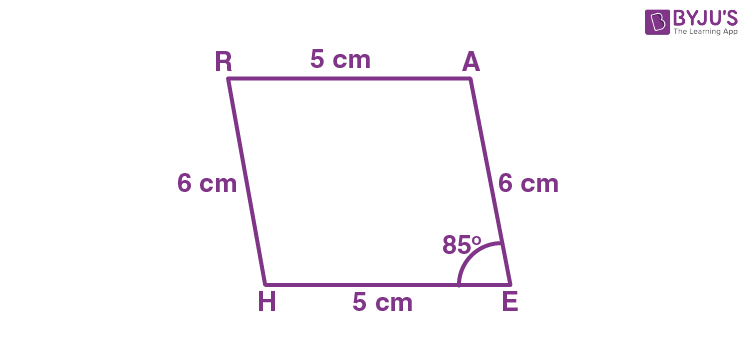Step (i): Draw a line segment HE of 5 cm and an angle of 85º at point E. As vertex A is 6 cm away from vertex E, cut a line segment EA of 6 cm from this ray.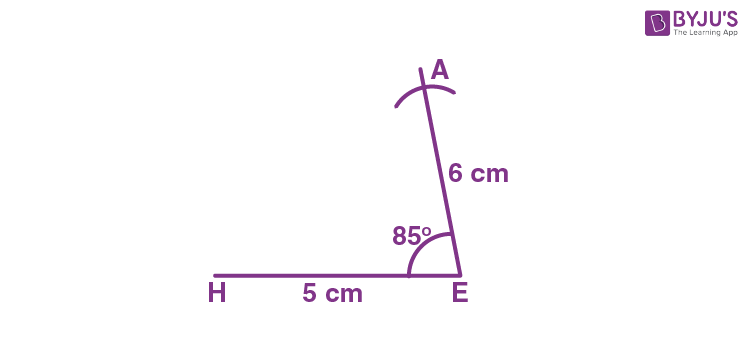Step (ii):  Vertex R is 6 cm and 5 cm away from vertex H and A respectively. By taking a radius as 6 cm and 5 cm, draw arcs from point H and A respectively. These will be intersecting each other at point R.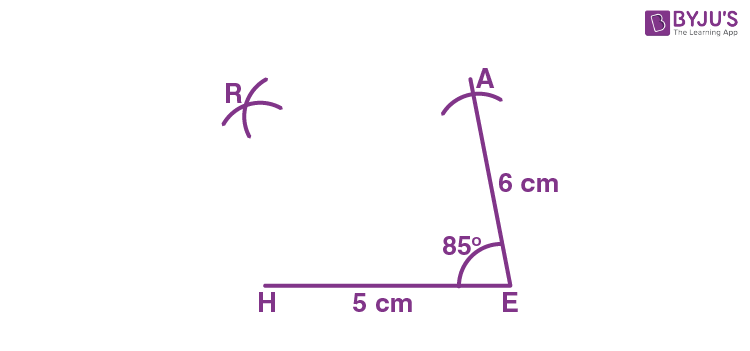Step (iii): Join R to H and A.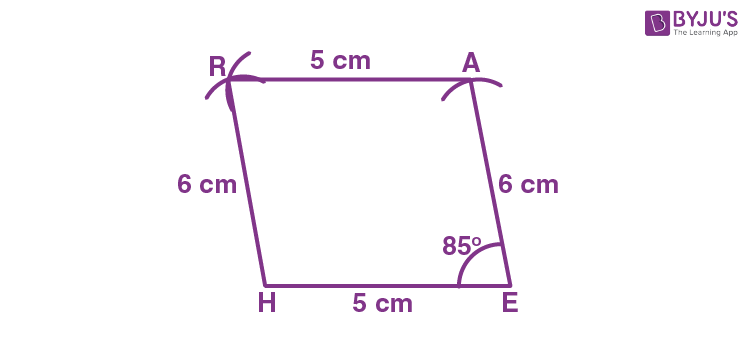(b) Yes, Early childhood education provides children to learn with diversity. This helps in creation of a better society.

Q.2) Divide the sum of

$$\begin{array}{l}\frac{7}{8}\end{array}$$
and
$$\begin{array}{l}\frac{15}{24}\end{array}$$

Solution:

Sum of 7/8 and 15/24:

(7/8) + (15/24)

= (21 + 15)/24

= 36/24

= 3/2

Difference of 7/8 and 15/24:

(7/8) – (15/24)

= (21 – 15)/24

= 6/24

= 1/4

Now, dividing the sum by difference,

(3/2)/ (1/4)

= (3/2) × 4

= 6

Q.3) The denominator of a rational number is greater than its numerator by 6. If the numerator is decreased by 1 and denominator is increased by 1, the number obtained is

$$\begin{array}{l}\frac{1}{3}\end{array}$$
. Find the rational number.

Solution:

Let x be the numerator of a rational number.

Then, denominator = x + 6

As per the given condition,

(x – 1)/(x + 6 + 1) = 1/3

(x – 1)/(x + 7) = 1/3

3(x – 1) = (x + 7)

3x – 3 = x + 7

3x – x = 7 + 3

2x = 10

x = 5

Therefore, the rational number = 5/(5 + 6) = 5/11

Q. 4) PJUM is a parallelogram. Its diagonal meet at point O. Find x and y.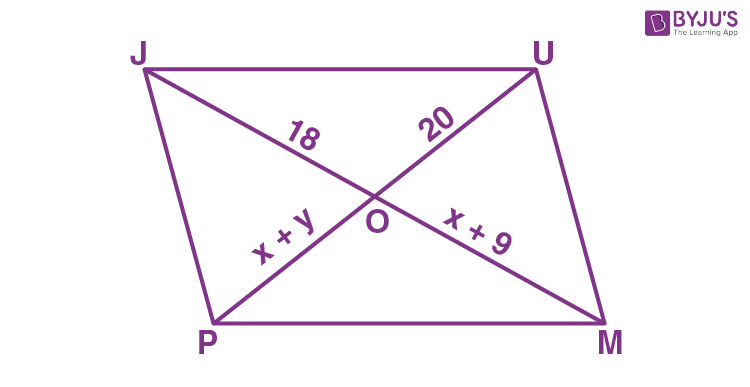Solution:

We know that the diagonals of a parallelogram bisect each other.

y + 9 = 18

y = 18 – 9

y = 9

Also, x + y = 20

Substituting y = 9,

x + 9 = 20

x = 20 – 9

x = 11

Therefore, x = 11 and y = 9.

Q. 5) Draw a histogram for the daily earnings of 40 drug stores in following table:

 Daily Earnings in Rupees 450-500 500-550 550-600 600-650 650-700 Number of Stores 16 10 8 4 2

Solution:

The class limits are represented along the x-axis and the frequencies along the y-axis on a suitable scale. The rectangles can be drawn to obtain the histogram for the given frequency distribution, taking class intervals as bases and the corresponding frequencies as heights. The histogram is given below: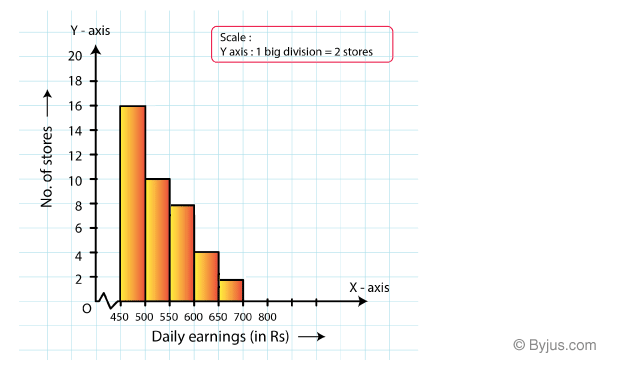Q. 6) Sanya took a loan of Rs. 80000 from a bank. If the rate of interest is 10% per annum, find the difference in amount she would pay after

$$\begin{array}{l}1\frac{1}{2}\end{array}$$
years, if the interest is compounded:

• a) Annually
• b) Half Yearly

Solution:

Given,

Principal amount = P = Rs. 80000

Rate of interest = r = 10%

(i) Compounded annually

Amount after 1 year = 80000 + 10% of 80000 = 80000 + 8000 = 88000

Interest for the next 6 months = 88000 x (10/2)% = 4400

Amount after one and half years = Rs. 88000 + Rs. 4400 = Rs. 92400

(ii) Compounded half-yearly

In this case, the rate of interest will become half and time will be 3 half years.

Thus, amount = 80000[1 + (5/100)]3

= 80000[1 + (1/20)]3

= 80000 × (21/20) × (21/20) × (21/20)

= 92610

Now, the difference = Rs. 92610 – Rs. 92400 = Rs. 210

Q. 7) Sarita borrowed Rs. 18000 from Vimla at 3% per annum simple interest for 2 years. If she had borrowed this sum at 3% per annum compound interest, what extra amount would she have to pay?

Solution:

Given,

P = Rs. 18000

Rate of interest = R = 3% p.a.

Time = 2 years

Simple interest = PTR/100

= (18000 × 2 × 3)/100

= 1080

Amount to be returned on simple interest = Rs. 18000 + Rs. 1080 = Rs. 19080

Amount to be returned on compound interest = P[1 + R/100]nt

= 18000[1 + (3/100)]2

= 18000 × (103/100) × (103/100)

= 19096.2

Difference = Rs.19096.2 – Rs. 19080 = Rs. 16.2

Sarita has to pay Rs. 16.2 extra if she had borrowed the amount on compound interest.

Q.8) Simplify and write the answer with positive exponents:

$$\begin{array}{l}\frac{3^{-5}\times 10^{-5}\times 125\times t^{4}}{5^{-7}\times t^{-2}}\end{array}$$

Solution:

$$\begin{array}{l}\frac{3^{-5} \times 10^{-5}\times 125 \times t^4}{5^{-7}\times t^{-2}}\\ = \frac{3^{-5} \times 5^{-5}\times 2^{-5}\times 5^3 \times t^4}{5^{-7}\times t^{-2}}\\ = 3^{-5} \times 5^{-5}\times 2^{-5}\times 5^3 \times t^4 \times 5^7 \times t^2\\ = 3^{-5} \times 5^{5}\times 2^{-5} \times t^{6}\\ = 6^{-5} \times 5^{5}\times t^{6}\\ = \frac{5^{5}\times t^{6}}{6^5}\end{array}$$

Q. 9) 500 people were stranded on an island they had enough food to last for 30 days. How long the food would last if there were 200 more people?

Solution:

Given,

Food last for 500 people = 30 days

Now, 200 more people joined.

500 people = 30 days

700 people = n

Here, the data follows an inverse proportion.

Thus, 500 × 30 = 700 × n

n = (500/700) × 30

n = 150/7 days

Q. 10) 6 persons could build a wall in 10 days. 3 persons left before the work started. How long it would take to build the wall now?

Solution:

Given,

6 people could build a wall in 10 days.

3 people left before the work started.

Now, the number of persons = 6 – 3 = 3

 Persons 6 3 Days 10 ?

This shows an inverse proportion.

Number of days required to build the wall = (6/3) × 10

= 20 days

Q. 11) Draw a graph for the following table of values, with suitable scale on the axis:

 Deposit(in Rs) 1000 2000 3000 4000 5000 Simple Interest(in Rs) 80 160 240 320 400

Solution:

We can draw a linear graph for the given data.

Take “Simple interest” on X-axis and “Deposit” on Y-axis.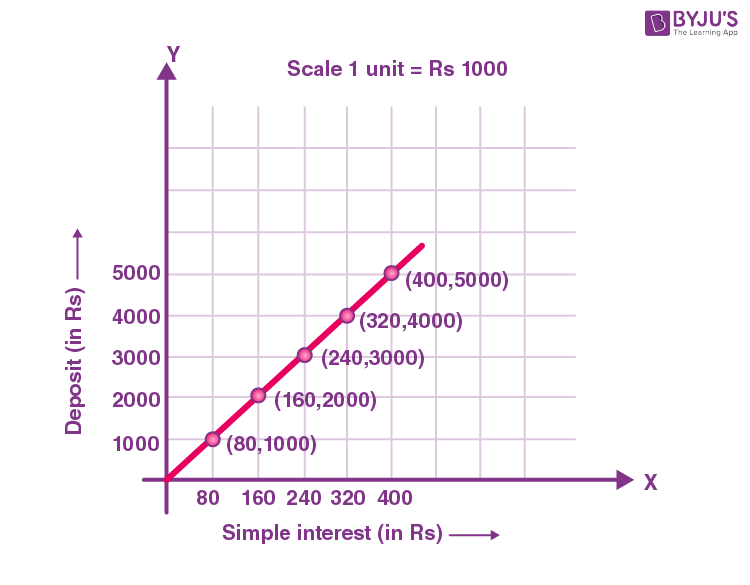Q.12) If each edge of a cube is doubled

• a) How many times will its surface area increase?
• b) How many times will its volume increase?

Solution:

Let “a” be the edge of a cube.

Surface area of cube with edge “a” = 6a2

Volume of cube = a3

New measure of the edge = 2a

(i) New surface area = 6(2a)2

= 6 × 4 × a2

= 4 × (6a2)

= 4 × original surface area

Thus, if the edge of a cube is doubled, then its surface area will be increased by 4 times.

(ii) New volume = (2a)3

= 8 × a3

= 8 × original volume

Thus, if the edge of a cube is doubled, then its volume will be increased by 8 times.

Q.13) A rectangular piece of paper 12 cm x 5 cm is folded without overlapping to make a cylinder of height 4 cm. Find the volume of the cylinder.

Solution:

Given that,

Length of the rectangular paper = 12 cm

Breadth of the rectangular paper = 5 cm

Circumference of circular part of cylinder = 2πr

Also, given that the paper is rolled along its length.

Circumference of circular part of cylinder = Length of the rectangular paper

2πr = 12 cm

2 × (22/7) × r = 12

r = (12 × 7)/44

r = 84/44

r = 21/11 cm

Height of cylinder = Breadth of the rectangular paper = h = 4 cm

Volume of cylinder = πr2h

= (22/7) × (21/11) × (21/11) × 4

= 45.82 cm3

Q. 14) Subtract the sum of 3a + 2b – c and 5b – 6a + 2ab from the sum of 9b -11ab and -4a + 21ab

Solution:

3a + 2b – c + 5b – 6a + 2ab

= (3a – 6a) + (2b + 5b) + 2ab

= -3a + 7b + 2ab….(i)

9b -11ab + (-4a + 21ab)

= 9b + (-11ab + 21ab) – 4a

= 9b + 10ab – 4a….(ii)

Subtracting (i) from (ii),

9b + 10ab – 4a – (-3a + 7b + 2ab)

= 9b + 10ab – 4a + 3a – 7b – 2ab

= 8ab – a + 2b

Q.15) In a cylindrical dome there are 16 pillars. The radius of each pillar is 35 cm and height is 7 m. Find the total cost of painting the curved surface area of all pillars at the rate of Rs. 8.50 per

$$\begin{array}{l}m^{2}\end{array}$$
.

Solution:

Given,

Radius of cylindrical pillar = r = 35 cm = 0.35 m

Height of the pillar = h = 7 m

Curved surface area of cylinder = 2πrh

= 2 × (22/7) × 0.35 × 7

= 15.4 m2

The curved surface area of 16 pillars = 16 × 15.4 m2 = 246.4 m2

Also, given that the cost of painting one m2 = Rs. 8.50

Total cost of painting the curved surface area of 16 pillars = 246.4 × Rs. 8.50 = Rs. 2094.4

Q.16) Water is pouring in a cuboidal reservoir at the rate of 60 liters per minute. If the volume of reservoir is 108

$$\begin{array}{l}m^{3}\end{array}$$
, find the number of hours it will take to fill the reservoir.

Solution:

Given, volume of reservoir = 108 m3

Rate of pouring water into cuboidal reservoir = 60 liters/minute

=  60/1000  m3 per minute {since 1 liter = (1/1000 )m3}

= (60 × 60)/1000 m3 per hour

Therefore, (60 × 60)/1000 m3 water filled in the reservoir in 1 hour.

Time taken for 1 m3 water to be filled in reservoir =  1000/(60 × 60) hours

Time taken for 108 m3 water to be filled in reservoir = (108 × 1000)/(60 × 60)  hours = 30 hours

Q.17) Praful reads 15 pages of a storybook in a day. Raju reads 10 pages of the same book in a day. How many days will Raju take to finish the fiction book if Praful finishes in 13 days?

Solution:

Given,

Number of pages read by Praful in a day = 15

Number of pages read by Raju in a day = 10

Number of days required for Praful to finish the book = 13

That means, total number of pages in the book = 15 × 13 = 195

Hence, the number of days required for Raju to finish the book = 195/10 = 19.5 days

Q.18) Find the least number that must be added to 9911 so as to get a perfect square. Also find the square root of perfect square obtained.

Solution:

Let us find the square root of 9911.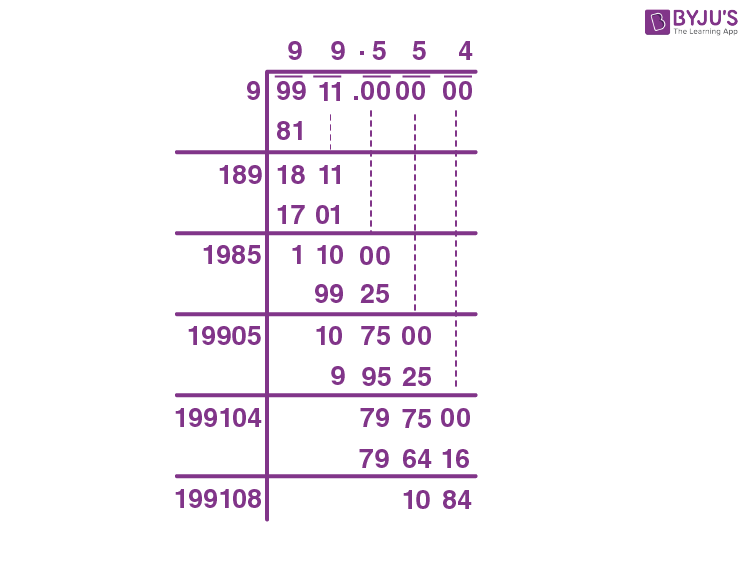Thus, the square of 100 will be the nearest perfect square number to 9911.

1002 = 10000

Subtracting 9911 from the square of 100,

10000 – 9911 = 89

Therefore, the least number to be added to 9911 so as to get a perfect square is 89.

Q.19) Plot the distance time graph for given values:

 Distance (in km) 125 150 175 200 Time (min.) 25 30 35 40

Solution:

Considering the distance on the vertical axis and time on the horizontal axis.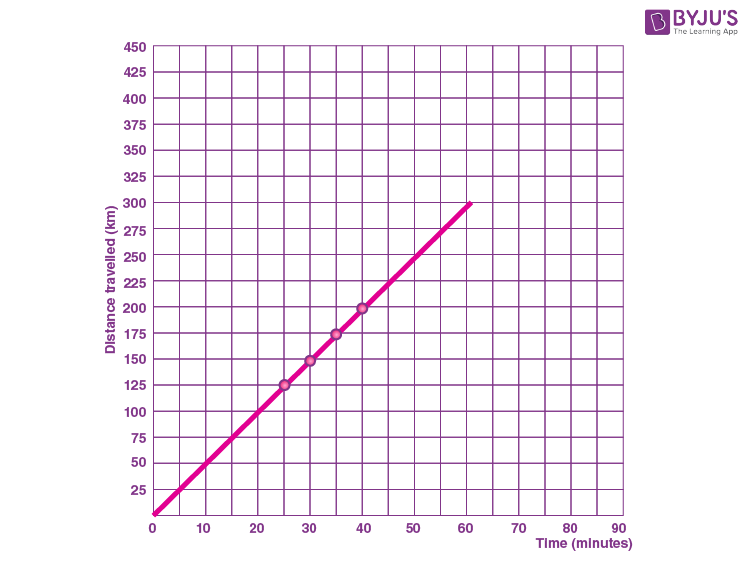Q.20) Factorise the expressions and divide them;

$$\begin{array}{l}lm(l^{4} – m^{4})\div l(l^{2}+m^{2})\end{array}$$

Solution:

lm(l4 – m4) ÷ l(l2 + m2)

= lm[(l2)2 – (m2)2]/ [l(l2 + m2)]

Using the identity a2 – b2 = (a + b)(a – b),

= [lm(l2 + m2)(l2 – m2)]/ [l(l2 + m2)]

= m(l2 – m2)

## More Articles for Class 8

1. Ritik singhania

Good